# Tunnel Effect

## Introduction

One of those cool effects used in oldskool demos is the Tunnel Effect. This effect shows a tunnel in which you fly while the tunnel rotates, seemingly in 3D. This tutorial will explain how to make one.

## How it works

An example tunnel looks like this, only in reality it animates: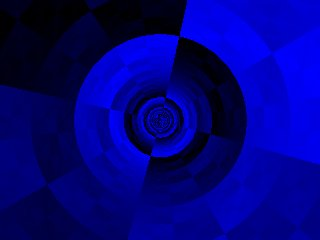The effect works as follows:

First, you need a texture, which is the texture of the sides of the tunnel.

The animation of the tunnel actually isn't calculated on the fly while the animation runs, but it's precalculated. These calculations are stored in two tables: one for the angle and one for the distance.

The distance table contains for every pixel of the screen, the inverse of the distance to the center of the screen this pixel has. This gives pixels of the center of the screen a very high value (those are very far away, as you can see on the picture above), while the pixels on the sides of the screen get a low value (these pixels represent parts of the tunnel close to the camera).

The angle table contains the angle of every pixel of the screen, where the center of the screen represents the origin.

Then after everything is precalculated the animation loop starts. This animation loop goes every frame, through every pixel (x,y), and then uses the angle and distance table to ask which texel of the texture it should draw at the current pixel. And, to get the animation, the values of the angle and distance table are shifted: shifting the angle table makes the tunnel rotate, while shifting the distance table makes you move forwards or backwards.

The texture has only a finite size, while the values gotten by the distance go up to infinity, and the values of the angle are periodic. When a value outside the texture is asked, modulo divide it through the size of the texture, this way we get the texture repeated over and over (but always smaller as it's closer to the center of the screen). You'll see this much better in the code.

## The code

This code creates a tunnel where you fly forward while the tunnel rotates, and the center of the tunnel always remains in the center of the screen. The code is made so that no matter what size the texture has, the effect will always have the same speed, and the texture is as big on the screen.

Here a few values are defined and buffers are created.

The texture array is the texture (duh). The bigger its size, the better the effect, I recommend making it at least 256*256 pixels, because it'll be as big as the screen, and the rotation goes very shaky if the texture is too small.

The distanceTable is the precalculated table for the inverse distance of every pixel, and the angleTable is the precalculated angle of every pixel. These have to be at least as big as the screen, but if you also want to move the center of the tunnel around on the screen, you'll have to make them bigger.

And the buffer array is used to draw the pixels to, so that the whole screen buffer can be drawn at once instead of using pset for every separate pixel.

 ```#define texWidth 256 #define texHeight 256 #define screenWidth 640 #define screenHeight 480 // Y-coordinate first because we use horizontal scanlines Uint32 texture[texHeight][texWidth]; int distanceTable[screenHeight][screenWidth]; int angleTable[screenHeight][screenWidth]; Uint32 buffer[screenHeight][screenWidth];```

Here the main function starts, and a blue XOR texture is generated (you can also load one from a bitmap instead).

 ```int main(int argc, char *argv[]) { screen(screenWidth, screenHeight, 0, "Tunnel Effect"); //generate texture for(int y = 0; y < texHeight; y++) for(int x = 0; x < texWidth; x++) { texture[y][x] = (x * 256 / texWidth) ^ (y * 256 / texHeight); }```

Next the buffers are generated. The distance buffer uses the formula of the inverse distance to the center of the screen (in 2D), multiplied by texHeight so that no matter which size the texture has, it's always as big. It's also modulo divided through texHeight so that the same texture is repeated all the time over the whole distance.

The angle buffer calculates the angle of the current pixel (the angle it has to the pixel in the center of the screen), by using the atan2 function. The atan2 function belongs to the <cmath> header, and returns the angle in radians of a given point by giving its x and y coordinate. It's divided through pi, so that the texture will be wrapped exactly one time around the tunnel.

The ratio variable is the ratio between the width and height the texture will be having on screen, or how long the texture stretches out in the distance.

 ``` //generate non-linear transformation table for(int y = 0; y < h; y++) for(int x = 0; x < w; x++) { int angle, distance; float ratio = 32.0; distance = int(ratio * texHeight / sqrt((x - w / 2.0) * (x - w / 2.0) + (y - h / 2.0) * (y - h / 2.0))) % texHeight; angle = (unsigned int)(0.5 * texWidth * atan2(y - h / 2.0, x - w / 2.0) / 3.1416); distanceTable[y][x] = distance; angleTable[y][x] = angle; }```

Then the animation loop starts.

The animation variable is set to the time in seconds, and will be used for shifting the tables for rotation and the moving.

Then for every pixel (x,y), the correct texel is gotten from the texture by using the tables, and shifted with the animation value. The modulo division through the width and height of the texture are to make sure we won't ask for a pixel outside the texture, but use the same texture tiled instead. Unsigned integers are used, because the values can become negative, and the modulo division only works correctly if they're unsigned instead, so that negative numbers will wrap around. Because of this, the texture width and height should be powers of 2, or they won't nicely tile. The animation values (shiftX and shiftY) are multiplied with the texture width and height to make the speed of the effect independent of the texture size, only of the time.

The animation of the distance and angle is also multiplied with a constant value (1.0 and 0.25 here), by changing these you can independently change the speed of the rotation, and of the moving forward.

The pixels of the screen are drawn to the buffer, and after all pixels are drawn, the buffer is put on screen, and the process restarts again for the next frame.

 ``` float animation; //begin the loop while(!done()) { animation = getTime(); //calculate the shift values out of the animation value int shiftX = int(texWidth * 1.0 * animation); int shiftY = int(texHeight * 0.25 * animation); for(int y = 0; y < h; y++) for(int x = 0; x < w; x++) { //get the texel from the texture by using the tables, shifted with the animation values int color = texture[(unsigned int)(distanceTable[y][x] + shiftX) % texWidth][(unsigned int)(angleTable[y][x] + shiftY) % texHeight]; buffer[y][x] = color; } drawBuffer(buffer); redraw(); } return(0); }```

This is what the tunnel looks like, but it also animates: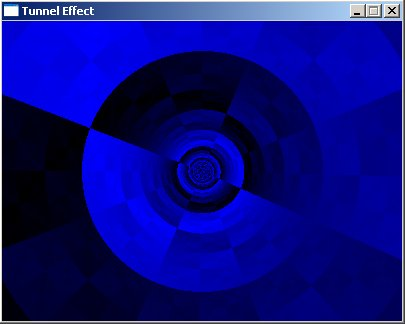## Better textures

Use this code instead of the code that generates the XOR pattern to load a texture:

 `loadBMP("textures/tunnel.bmp", texture, texWidth, texHeight);`

Here are two textures from Unreal Tournament 2003 applied to the tunnel: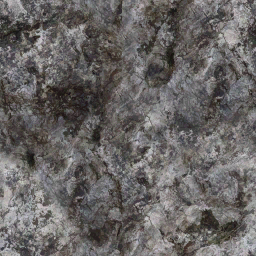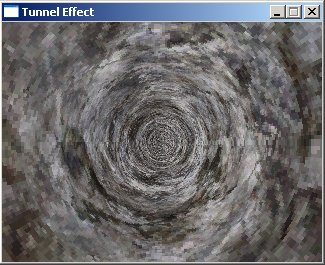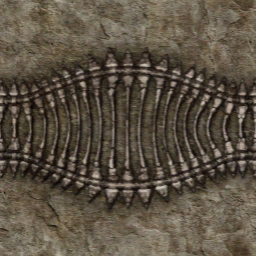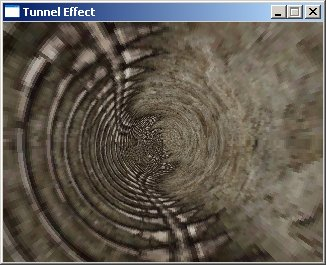## Looking Around

Because you keep moving forward all the time, the effect becomes boring fast. To make it a bit more interesting, the camera can be made to look around. To do this, simply move the center of the tunnel around, it then seems as if the camera rotates. If you want to move the center of the tunnel around, bigger precalculated buffers are needed. Here's a modified version of the code that'll do this. The bold parts are new or changed, and the comments should explain it.

 ```#define texWidth 256 #define texHeight 256 #define screenWidth 400 #define screenHeight 300 int texture[texWidth][texHeight]; //Make the tables twice as big as the screen. The center of the buffers is now the position (w,h). int distanceTable[2 * screenWidth][2 * screenHeight]; int angleTable[2 * screenWidth][2 * screenHeight]; int buffer[screenWidth][screenHeight]; int main(int argc, char *argv[]) { screen(screenWidth, screenHeight, 0, "Tunnel Effect"); loadBMP("textures/tunnel.bmp", texture, texWidth, texHeight); //generate non-linear transformation table, now for the bigger buffers (twice as big) for(int y = 0; y < h * 2; y++) for(int x = 0; x < w * 2; x++) { int angle, distance; float ratio = 32.0; //these formulas are changed to work with the new center of the tables distance = int(ratio * texHeight / sqrt(float((x - w) * (x - w) + (y - h) * (y - h)))) % texHeight; angle = (unsigned int)(0.5 * texWidth * atan2(float(y - h), float(x - w)) / 3.1416); distanceTable[y][x] = distance; angleTable[y][x] = angle; } float animation; //begin the loop while(!done()) { animation = getTime() / 1000.0; //calculate the shift values out of the animation value int shiftX = int(texWidth * 1.0 * animation); int shiftY = int(texHeight * 0.25 * animation); //calculate the look values out of the animation value //by using sine functions, it'll alternate between looking left/right and up/down //make sure that x + shiftLookX never goes outside the dimensions of the table, same for y int shiftLookX = w / 2 + int(w / 2 * sin(animation)); int shiftLookY = h / 2 + int(h / 2 * sin(animation * 2.0)); for(int y = 0; y < h; y++) for(int x = 0; x < w; x++) { //get the texel from the texture by using the tables, shifted with the animation variable //now, x and y are shifted as well with the "look" animation values int color = texture[(unsigned int)(distanceTable[x + shiftLookX][y + shiftLookY] + shiftX) % texWidth] [(unsigned int)(angleTable[x + shiftLookX][y + shiftLookY]+ shiftY) % texHeight]; buffer[y][x] = color; } drawBuffer(buffer); redraw(); } return(0); }```

Here for example it's looking to the bottom left, so the center of the tunnel is in the top right of the screen, but the looking direction changes all the time while the effect runs: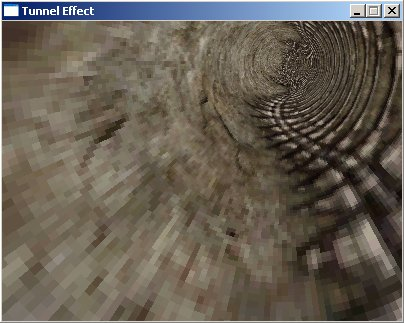If you're interested, because two sine functions are used to look around, one for the looking in the x direction, and one for the looking in the y direction, the look-around movement (or the path the center of the tunnel follows on the screen) has the shape of a Lissajous figure.

Last edited: 2004